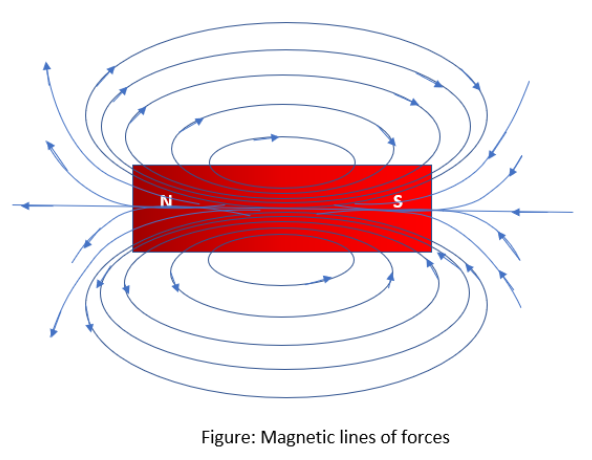# Properties of magnetic lines of force are:A) The imaginary lines which represent the direction of the magnetic field, are known as magnetic lines of force.B) Magnetic lines of force are closed curves.C) Outside the magnet their direction is from north pole to south pole and inside the magnet these are from south to north pole.D) AllVerified
161.5k+ views
Hint: Magnetic field lines are also known as magnetic lines of force. It is actually imaginary lines to easily learn about electromagnetism. It will be easy to understand the properties of field lines if you draw the magnetic lines of force for a bar magnet.

Complete step by step answer:Magnetic field lines of a bar magnet are shown in this diagram. These magnetic lines of force imply the presence of magnetic force around the magnet. It has several properties as follows.
Magnetic field lines originate from the north pole and terminate at the south pole outside of a magnet.
They form continuous closed curves.
The direction of the magnetic field lines inside the magnet is from south pole to north pole.
The magnetic field lines never intersect each other. If they intersect there will be two directions for the magnetic field. It is not allowed.
The density of magnetic lines decreases as the distance between the poles increases.
Magnetic strength increases at the dense magnetic field lines.
So, the option (D) is correct.
Additional information: Magnetic lines of force are imaginary lines to represent their magnetic field. It gives the force direction. It helps to understand the density of the magnetic field lines; hence magnetism will be easier to understand. These can be easily demonstrated by using a bar magnet and iron powder. At the poles of the magnet, the magnetic field will be stronger. Magnetic flux is the term used to describe the number of lines of force passing through the unit area.
Since the magnetic field is a vector quantity, we need two aspects to measure it and describe it. Magnetic strength and direction are the two aspects. Direction can find out with a magnetic compass, because it will align the same direction of field lines. Measuring magnetic field strength is a little bit difficult. Magnetoresistance measurements are available now for the measurements of small magnetic fields. Tesla (T) is the unit of magnetic field. It is defined as the amount of force required to move a charge due to field. Gauss (G) is also used as the unit of magnetic field.
$1\text{ T = 1}{{\text{0}}^{4}}\text{ G}$

Note: Commonly the collective answer will be the last option. So, you have to go through all the options. Otherwise you will choose the first option and will get negative marks. In this question all answers are correct. It is better to learn the direction of field lines.